# 编译原理

• 编译：将高级语言 翻译 成汇编语言或机器语言的过程。
• 编译器结构：
• 词法分析，扫描识别源程序，得到词法单元 token 序列。
• 语法分析，构造语法分析树。
• 语义分析，收集标识符的属性信息，语义检查。
• 中间代码生成，三地址码，语法树等结构
• 机器无关代码优化
• 目标代码生成
• 机器相关代码优化

## 一、语言&文法&词法分析

### 1.0 基本概念

• 基本符号
• Σ：字母表，形式符号的集合
• ε：空串
• |w|：字符串w的长度
• 基本运算
• 连接，如x, y为串，xy表连接
• 幂运算，即对串的重复
• * 闭包，同正则表达式中 *，可重复0~∞次
• + 闭包，同正则表达式中 +，可重复1~∞次

• 文法，$G=(V_T,V_N,P,S)$
• $V_T$：终结符集合
• $V_N$：非终结符集合，非终结符是用来表示语法成分的符号，有时也称为“语法变量”
• $V_T∩V_N=∅$
• $V_T∪V_N$：文法符号集
• $P$：产生式集合
• $S$：开始符号
• 文法的通常 符号表示
• 终结符：a, b, c
• 非终结符：A, B, C
• 文法符号：X, Y, Z
• 终结符号串：u, v…z
• 文法符号串：α, β, γ

• 推导
• 用产生式的右部替换产生式的左部
• $α_0⇒^nα_n$，表示 n 步推导
• 归约
• 用产生式的左部替换产生式的右部

$$L(G)=\{w|S⇒^∗w,w∈V^∗_T\}$$

• 语言上的运算：
• 并，$L∪M=\{w|w∈L∨x∈M\}$
• 连接，$LM=\{w_1w_2|w_1∈L∧w_2∈M\}$
• *闭包，$L^∗=L^0∪L^1∪L^2…$

• 句型
• 如果 $S⇒^∗α，α∈(V_N∪V_T)^∗$，则称 α 为 G 的一个句型。
• 句子
• 如果 $S⇒^∗w，w∈V^∗_T$，则称 w 为 G 的一个句子。
• 句子是不包含非终结符的句型

### 1.1 Chomsky 文法分类

• 0 型文法，无规则文法
• $∀α→β∈P$，α 中至少包含一个非终结符
• 1 型文法，上下文有关文法，Content-Sensitive Grammar
• $∀α→β∈P$，|α|≤|β|
• CSG 中不包含 ε-产生式
• 2 型文法，上下文无关文法，Content-Free Grammar
• $∀α→β∈P$，α∈VN
• 即每个产生式左部为非终结符
• 3 型文法，正则文法，Regular Grammar
• 右线性文法，$A→wB$ 或 $A→w$
• 左线性文法，$A→Bw$ 或 $A→w$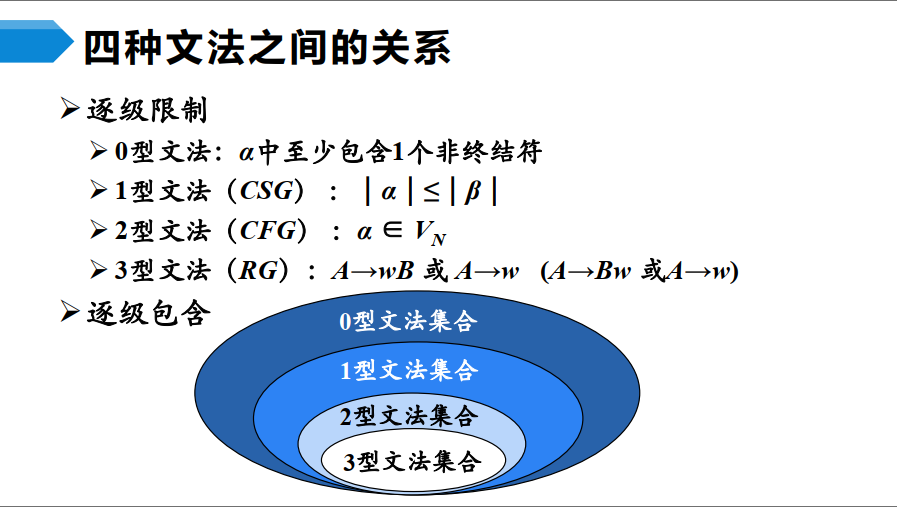### 1.2 CFG 的分析树

• 根节点，标号为文法开始符号
• 内部节点表示对一个产生式的应用
• 叶节点的标号既可以是非终结符，也可以是终结符
• 从左到右排列叶节点得到的符号串，称为这棵树的产出或边缘

⇒ 直接短语一定是某产生式的右部
⇒ 产生式的右部不一定是 给定句型 的直接短语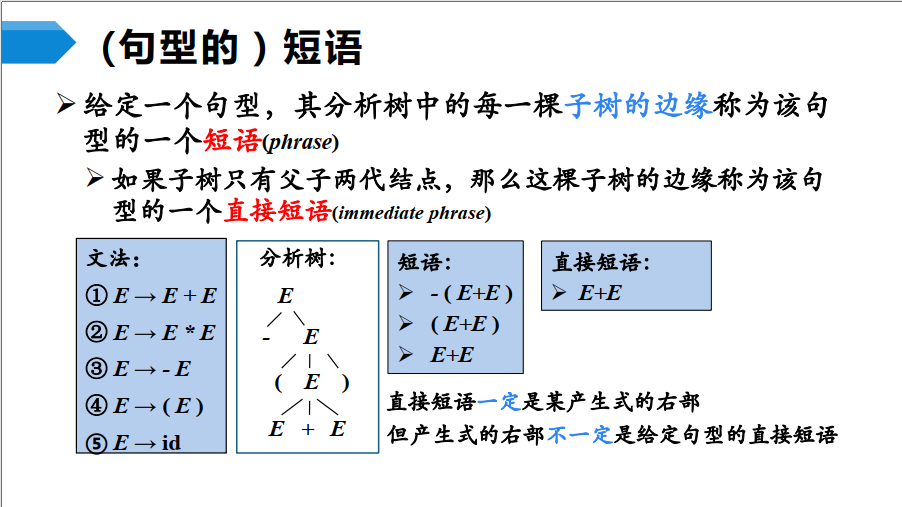### 1.3 正则表达式

• 3型（正规）文法
• 有限自动机
• 正规表达式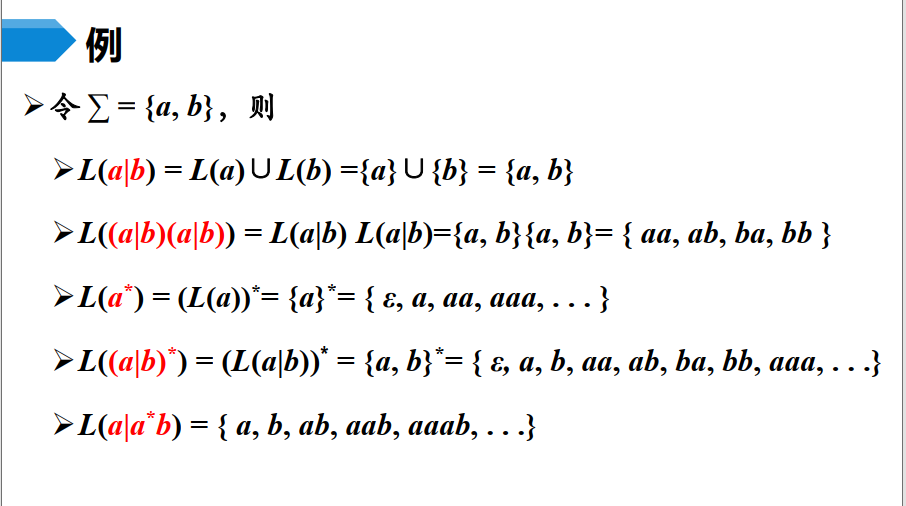### 1.4 有限自动机

$$M=(S,Σ,δ,s_0,F)$$

$$M=(S,Σ,δ,s_0,F)$$
$S$：有限状态集
$Σ$：有限输入符号集
$δ$：转移函数
$s_0$：开始状态，$s_0∈S$
$F$：接受状态（或终止状态）集合

• 表示方式：
• 转移图
• 非空有限状态，用圆圈表示
• 终态，双圆圈表示
• 开始状态，start箭头标出
• 转移函数，用带箭头圆弧表示
• 非空的有限输入符号，标在圆弧旁边
• 转移表
• 定理：L是某个 DFA 的语言，当且仅当L也是某个 NFA 的语言。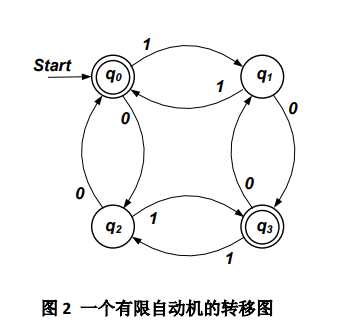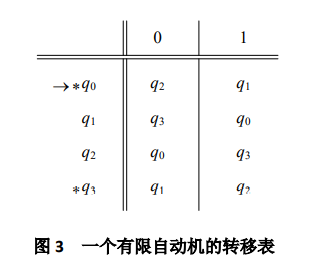## 二、自顶向下语法分析

### 2.0 概述

_自顶向下的分析_，可以看成是从文法开始符号 S 推导出词串 w 的过程。

• 两类非确定性
• 每一步的推导中，对哪一个非终结符进行展开
• 若选定的非终结符是多个产生式的左部，如何选择

_最左推导_，总是选择每个句型的最左非终结符进行替换

• 递归下降分析（自顶向下语法分析的通用形式）
• 由一组过程组成，每个过程对应一个非终结符
• 同一非终结符的多个候选式存在共同前缀，将导致回溯现象，由此引出以下的分析
• 预测分析
• 是递归下降分析的一个特例，通过输入中向前看固定个数（通常为1）符号来选择正确的 A-产生式
• 可以对某些文法构造出向前看 k 个输入符号的预测分析器，该类文法有时也称为 LL(k)文法类
• 预测分析不需要回溯，是一种确定的自顶向下分析方法
void A(){
选择一个 A 产生式
for(i to k){
if (xi 是一个非终结符)
调用过程 xi
else if (xi 为当前的输入符号 a)
读入下一个输入符号
else
发生错误
}
}


• 含有 $A→Aα$ 形式的文法称为是 直接左递归
• 如果一个文法中有一个非终结符 A 使得对某个串 α 存在一个推导 $A⇒^+Aα$，那么这个文法就是 左递归
• 左递归文法会使递归下降分析器陷入无限循环
• 经过两步或两步以上推导产生的左递归称为是间接左递归的

### 2.1 LL（1）分析⭐

$LL(1)$分析（自顶向下预测分析）

• First 集合
• 一个句型 α 若可以推导出另一个以终结符 a 开头的句型，那么 a 属于 $First(α)$；若 α 可以推导出 ε，那么 ε 属于 $First(α)$
• 即可推导的前缀（所有串首终结符）的集合
• Follow 集合
• 可能在某个句型中紧跟在 A 后面的终结符 a 的集合。若 G 中存在一个以 $X$ 结尾的句型，那么 # 属于 $Follow(X)$
• # 代表输入单词符号序列的结束符
• 算法：
• 初始时，置 $Follow(S)=\{\#\}$；对其它 $X∈V_N$，置 $Follow(X)=∅$
• 依次考虑每一个右端含有非终结符的产生式
• 在末情况下，
$$follow1=follow1∪follow2$$
• 不在末，
$$follow1=follow1∪（first2−\{ε\}）$$
• Select 集合
• 产生式 $A→β$ 的可选集是指可以选用该产生式进行推导时对应的输入符号的集合，记为 $SELECT(A→β)$
• 即可选用该产生式进行推导时，对应的终结符集合
1. $$SELECT(A→aβ)=\{a\}$$
2. $$SELECT(A→ϵ)=FOLLOW(A)$$
3. $$如果ϵ∉FIRST(α),那么SELECT(A→α)=FIRST(α)$$
4. $$如果ϵ∈FIRST(α),那么SELECT(A→α)=(FIRST(α)−\{ϵ\})∪FOLLOW(A)$$

### 2.2 程序设计

$LL(1)$ 分析程序的设计（参考 lecture 2）：

void ParseFunction()
{
MatchToken(T_FUNC); // T_FUNC 为匹配终结符 FUNC 的单词符号
MatchToken(T_ID); // T_ID 为匹配终结符 ID 的单词符号
MatchToken(T_LPAREN); //T_LPAREN 为匹配终结符 ’(’ 的单词符号
ParseParameterList();
MatchToken(T_RPAREN); // T_RPAREN 为匹配终结符 ’)’ 的单词符号
ParseStatement();
}

void MatchToken(int expected)
{
{
printf("syntax error \n"); //若不匹配，则报告出错信息，跳出
exit(0);
}
else //若匹配， 消费掉当前单词符号
}

void ParseA()
{
case PS(A→u1):
…… /*根据 u1 设计的分析过程*/
break;
case PS(A→u2):
…… /*根据 u2 设计的分析过程*/
break;
...
case PS(A→ un):
…… /*根据 un 设计的分析过程*/
break;
default:
printf("syntax error \n");
exit(0);
}
}


S → AB
A → aA | ε
B → bB | ε

void ParseS( ) // 主函数
{
ParseA( );
ParseB( );
}

void ParseA( )
{
{
case ′a′ :
MatchToken(‘a’ );
ParseA();
break;
case ′ b′, ′#′ :
break;
default:
printf("syntax error \n")
exit(0);
}
return A_num;
}

void ParseB( )
{
case ′b′ :
MatchToken(‘b’ );
ParseB( );
break;
case ′#′ :
break;
default:
printf("syntax error \n");
exit(0);
}
}

void Match_Token(int expected)
{
{
printf("syntax error \n")
exit(0);
}
else
}


### 2.3 预测分析表

other

• 消除左递归
• 直接左递归：文法中有形如 P→Pα 的产生式，α 为文法符号串
• 间接左递归：相应地，n>0
• 提取左公因子
• 字面意思，对相同左部的产生式提取
• 出错处理

## 三、自底向上语法分析

### 3.1 移进-归约分析

• 基本思想：借助一个栈和一个有限状态的分析引擎，根据如下动作以进入新状态
• Reduce: 依确定的方式对位于栈顶的短语进行归约。
• Shift: 从输入序列移进一个单词符号。
• Error: 发现语法错误，进行错误处理/恢复。
• Accept：分析成功。
• 栈内符号串 + 剩余输入 = “规范句型”
• 语法分析动作冲突
• 移进−归约冲突
• 到达一个不能确定下一步应该移进还是应该归约的状态。
• 归约−归约冲突
• 到达一个可以对多个短语进行归约的状态。

### 3.2 LR分析

L，代表从左向右扫描输入的单词序列
R，代表产生的是最右推导

LR分析 是一种表驱动的移进-归约分析

• LR 分析表
• ACTION表
• 在栈顶状态为k，当前输入符号为a时应该做什么
• si（Shift, 移进）, rj（Reduce, 归约）, acc, err
• GOTO表
• 依产生式归约，并进行状态转移

### 3.3 LR（0）分析

• 增广文法
• 如果 G 是一个以 S 为开始符号的文法，则 G 的增广文法 G' 就是在 G 中加上新开始符号 S' 和产生式 S' → S 而得到的文法，

• 活前缀
• 一个活前缀是某一右句型的前缀，它不超过该右句型的某个句柄
• 项目，描述了句柄识别的状态，每个产生式对应若干个项目
• 移进项目
$$S′→α.aβ$$
• 待约项目
$$S′→α.Bβ$$
• 归约项目
$$S′→α.$$
• 接受项目
$$S′→S.$$
• 后继项目，同属于一个产生式的项目，但圆点的位置在后一个位。

$LR(0)FSM$（有限状态自动机）的状态是一个 $LR(0)$ 项目集 $I$ 的闭包。

？接受项目不与同状态下的移进项目冲突

### 3.4 SLR（1）分析

SLR（1）分析，向前查看一个符号可解决冲突

• 如果每一状态的所有 归约项中要归约的非终结符的 Follow 集 互不相交， 则可以解决归约−归约冲突。
• 如果每一状态的所有 归约项中要归约的非终结符的 Follow 集 与该状态所有 移进项目要移进的符号集 互不相交，则可以解决移进−归约冲突。

SLR（1） 分析表的构造也基于LR（0） FSM，在SLR（1） 分析表中， ACTION 表的归约表项只适用于相应非终结符Follow 集中的输入符号

### 3.5 LR（1）分析

• LR（1）项目是在 LR（0）项目基础上增加一个终结符，称为向前搜索符
• 对于归约项目，下一个输入为该终结符时，才可归约
• 对于其它项目，向前搜索符只起到信息传递的作用

## 四、语法制导得语义计算基础

### 4.0 概述

• 语义分析要解决的问题
• 如何表示语义信息
• 文法符号（语法信息）->（设置） 语义属性
• 如何计算语义信息（语义属性）
• 产生式（语法规则）->（关联） 语义规则
• 语义属性
• 综合属性
• 非终结符，由子节点或本身的属性计算
• 终结符，词法值
• 继承属性
• 非终结符，由子节点或本身的属性计算
• 终结符，没有继承属性

### 4.2 语义计算

• S-属性文法
• 只包含综合属性
• L-属性文法
• 可以包含综合属性，也可以包含继承属性

## 五、代码优化和目标代码生成

### 5.0 概述

• 输入：某一种中间代码以及符号表等信息
• 输出：特定目标机或虚拟机的目标代码

• 基本块
• 程序中一个顺序执行的语句序列，其中只有一个入口语句和一个出口语句。
• 流图
• 可以为构成程序的基本块增加控制流程信息， 方法是构造一个有向图， 称之为流图或控制流图。
• 流图中，某一个基本块运行之后可以到达的所有基本块是该基本块的 后继基本块，可以直接运行并到达某一个基本块的所有基本块是该基本块的 前驱基本块
• 循环
• 支配节点集，如果从流图的首结点出发，到达 n 的的任意通路都要经过 m, 则称 m 支配 n, 或 m 是 n 的 支配结点
• 回边，有向边，能回到支配结点

in [S]来定义 out [S]，这种数据流称为是向前流，相应的数据流方程（如 5-1）称为正向数据流方程。

• 到达-定值数据流分析
• in 到达基本块 B 入口处（入口语句之前的位置） 的各个变量的所有定值点集合。
• out 到达 B 出口处（紧接着出口语句之后的位置）的各个变量的所有定值点集合。
• gen B 中定值的并能够到达 B 出口处的所有定值点集合， 即 B 所“产生” 的定值点集合。
• kill B 所“杀死” 的定值点集合。
• out = gen U （in - kill）

• livein B 入口处为活跃的变量的集合。
• liveout B 的出口处的活跃变量的集合。
• def B 中定值的且定值前未曾在 B 中引用过的变量集合。
• liveuse B 中被定值之前要引用的变量集合。
• livein = liveout U （liveout - def）

## 六、实验课

1.词法分析器自动构造工具JFLEX，S1词法分析器自动构造 √
2.词法分析器自动构造工具YACC，S1词法分析器自动构造 √
3.基础实验项目：表达式计算器Jflex/Yacc实现 √
4.J1_指令 √
5.实验项目PA1：Step1 S1编译器Jflex/Yacc实现 √
6.实验项目PA2：Step2 S2编译器实现 √
7.实验项目PA3：Step3 S3编译器实现；Step4 S4编译器实现 √

jflex 是一个 词法分析 的生成器，生成 Java 写的词法分析器，文件扩展名为 .l

yacc 是 Unix 系统上的一个 LALR（1）语法分析 生成器。yet another compiler compiler，文件扩展名为 .y

yacc -J S1ly.y
jflex S1l.l
javac Parser.java
javac Yylex.java
java Parser S1
a S1.a


## other

• 平时（40%）
• 书面作业（10%）
• 上机作业+出勤（15%）
• 实验大作业（15%）
• 期末（60%）

2021.7.7 考试结束，多写题

目录